# Predict the normal boiling point of hydrogen peroxide (H202) from the following data:AH°, kJ/molAS°, J/(mol•K)H2O2(1)|-187.8110233|-136.3H2O2(g)

Question
4 views

Tb =  ______ºC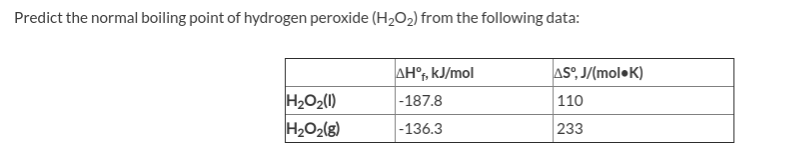help_outlineImage TranscriptionclosePredict the normal boiling point of hydrogen peroxide (H202) from the following data: AH°, kJ/mol AS°, J/(mol•K) H2O2(1) |-187.8 110 233 |-136.3 H2O2(g) fullscreen
check_circle

Step 1

Given data,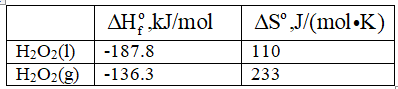When hydrogen peroxide (H2O2) starts boiling, liquid hydrogen peroxide (H2O2) change into gas hydrogen peroxidThe enthalpy and entropy are related to each otherwise equation shown below.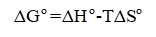Step 2

At boiling point, amount of liquid hydrogen peroxide (H2O2) change into gas is equal to the amount of gas hydrogen peroxide (H2O2) change into liquid. Therefore, liquid hydrogen peroxide (H2O2) is in equilibrium with gas hydrogen peroxide (H2O2) and ΔGo = 0.

Therefore,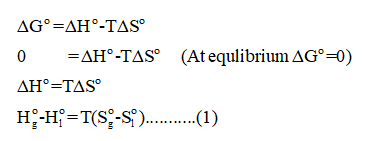Step 3

Substitute the given data in equation (1).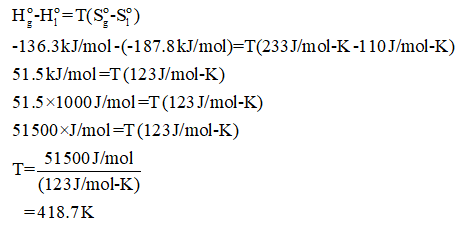The conversion from K to oC to is shown below.

...

### Want to see the full answer?

See Solution

#### Want to see this answer and more?

Solutions are written by subject experts who are available 24/7. Questions are typically answered within 1 hour.*

See Solution
*Response times may vary by subject and question.
Tagged in